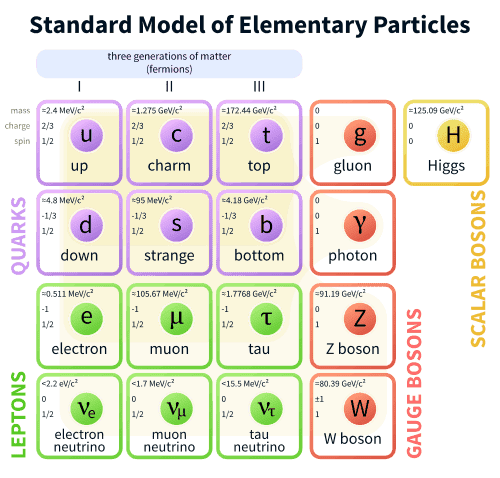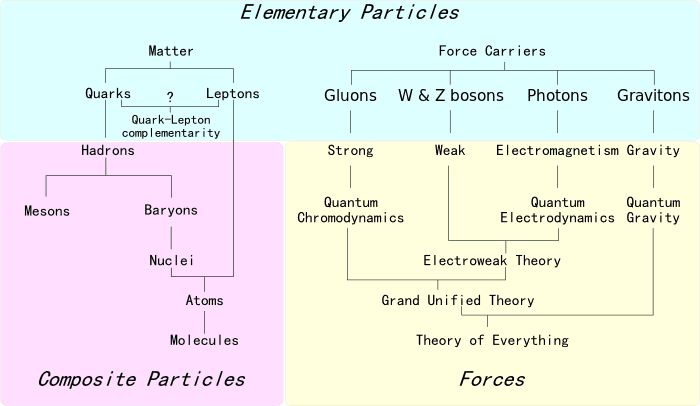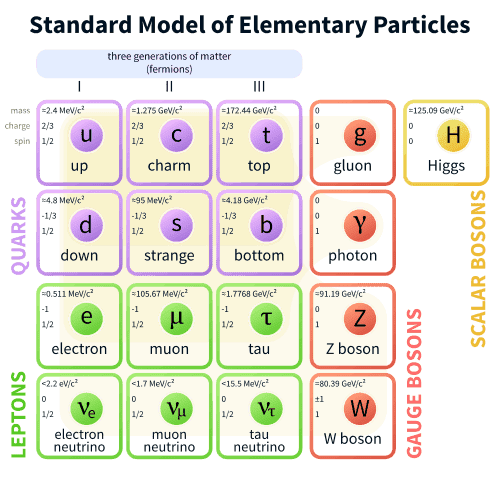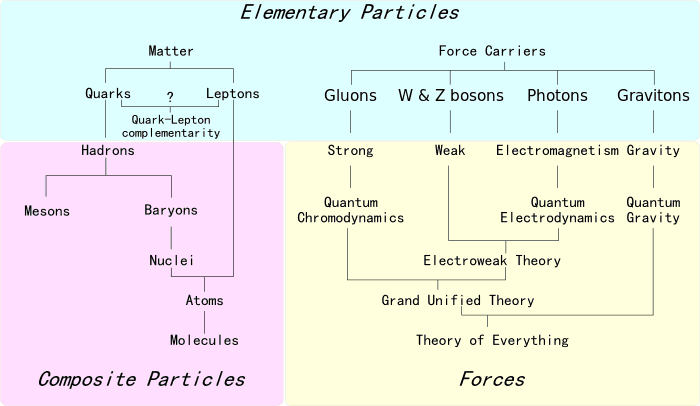# Neutrino Oscillations for Dummies

Gold Member
Neutrino Oscillation for Dummies

If someone has the time to answer these questions, it would be much appreciated.
• According to Wikipedia http://en.wikipedia.org/wiki/Neutrino_oscillation" [Broken] is of "interest since observation of the phenomenon implies that the neutrino has a non-zero mass". Does this mean that 100% confirmed oscillation unconditionally 'gives' mass to neutrinos? Or is there still a possibility for mass-less neutrinos to oscillate?

• I see users in this thread talking about neutrinos changing flavor thru flight, but no measurement is done, right? And 'all' we got before this is the QM wavefunction and a superposition of the three neutrino states, before the measurement, right?

• AFAICT, there’s a 'phase shift' between the 3 flavors in the wavefunction, and that the traveling distance is what finally sets the probabilities for measuring one flavor, correct?

• If neutrinos have mass and are only left-handed, that creates 'problems' with the Standard Model and the Higgs mechanism (which need both left- and right-handed versions of the fermion to function), right? But then I read somewhere that "neutrinos are mostly left-handed"...? Has right-handed neutrinos been detected?

• What’s the main reason neutrinos interact very weakly with 'ordinary' matter? Is it the 0 charge, or the tiny mass?

• If right-handed neutrinos exist, do they interact with the W and Z bosons?

• If neutrinos have mass, are they affected by gravity?

• [Just curiosity, nothing else]
If it’s confirmed that the difference in mass between the neutrino and other fermions is 500,000 times, and we know that the difference between gravity and the other three fundamental interactions is > 1025; could there be a 'relation' between these two very large 'gaps'... (to the other 'stuff')...?Thanks

Last edited by a moderator:

## Answers and Replies

Neutrino Oscillation for Dummies

If someone has the time to answer these questions, it would be much appreciated.
• According to Wikipedia http://en.wikipedia.org/wiki/Neutrino_oscillation" [Broken] is of "interest since observation of the phenomenon implies that the neutrino has a non-zero mass". Does this mean that 100% confirmed oscillation unconditionally 'gives' mass to neutrinos? Or is there still a possibility for mass-less neutrinos to oscillate?

• I see users in this thread talking about neutrinos changing flavor thru flight, but no measurement is done, right? And 'all' we got before this is the QM wavefunction and a superposition of the three neutrino states, before the measurement, right?

• AFAICT, there’s a 'phase shift' between the 3 flavors in the wavefunction, and that the traveling distance is what finally sets the probabilities for measuring one flavor, correct?

• If neutrinos have mass and are only left-handed, that creates 'problems' with the Standard Model and the Higgs mechanism (which need both left- and right-handed versions of the fermion to function), right? But then I read somewhere that "neutrinos are mostly left-handed"...? Has right-handed neutrinos been detected?

• What’s the main reason neutrinos interact very weakly with 'ordinary' matter? Is it the 0 charge, or the tiny mass?

• If right-handed neutrinos exist, do they interact with the W and Z bosons?

• If neutrinos have mass, are they affected by gravity?

• [Just curiosity, nothing else]
If it’s confirmed that the difference in mass between the neutrino and other fermions is 500,000 times, and we know that the difference between gravity and the other three fundamental interactions is > 1025; could there be a 'relation' between these two very large 'gaps'... (to the other 'stuff')...?Thanks

Okay, let's try these in order.

• To get oscillations what's actually necessary is that the neutrinos have non-degenerate mass states. Specifically, the oscillation probabilities will depend on $\Delta m^2$. So, if two states have the same mass, there will be no oscillation between them. That means that to get the observed number of independent oscillations observed (i.e. more than one), no more than one of the three neutrino mass states can have $m=0$.
• Yes. At any time, the flavor wave function is a superposition of the three gauge eigenstates (to some approximation - to really do it right, I think you have to think in terms of density matrices); and, it's the parameters of that superposition that oscillate.
• Approximately. But, the usual treatment assumes an exact energy and momentum for the neutrino. Since particles are actually created as wavepackets with some dispersion in energy and momentum, the pathlength part of the effect should get washed out if the neutrino source is extremely distant.
• I don't know that "problem" is quite the right way to put this. No matter what you're going to have to add something to the standard model in order to get neutrino masses. The only real question is what that will be and whether the Higgs will actually be involved at all. There are two pretty standard ideas about this (called the Type I and Type II see-saw mechanisms, respectively). In Type I, there are right-handed neutrinos which have the usual sort of coupling to the lepton doublet and the Higgs, but which also have a very large Majorana mass of their own. (Because they're uncharged under all gauge interactions in the theory, they can be their own antiparticles, which allows the definition of this mass term in a fully consistent way.) Then, when EW symmetry is broken a small mixing between the left-handed neutrinos and the heavy left-handed anti-neutrinos is induced, which results in a light state with mass $~\frac{m_{SM}^{\phantom{SM}2}}{M_{Majorana}}$, where $m_{SM}$ is the mass that EW symmetry breaking would create were there no Majorana mass present. In Type II, the lepton doublet couples to a new scalar triplet which has a potential such that EWSB gives it a small vacuum value that interacts with the left-handed neutrino in such a way as to generate a Majorana mass for the neutrino. Either way, none of the new states implied have been observed; but, in Type I the new states are extremely heavy and in Type II they tend to be quite weakly coupled. So, it's not guaranteed that anything should have been seen yet.
• It's the lack of net electric or color charges. The mass is incidental to this.
• Purely right-handed neutrinos will not interact with the W and Z at all. The post-mixing heavy neutrinos of the Type I see-saw will interact with the W and Z; but, the interaction strengths will be tiny.
• Neutrinos are affected by gravity even have they have no mass at all. Gravity couples to all forms of energy, momentum, pressure, etc.
• Amazingly enough, the gap between the neutrino and other fermion masses is actually quite a bit too small to be explained easily by gravitational effects. The only models I know of that relate the various hierarchies at all have to use extra dimensions to do so.

Last edited by a moderator:
Gold Member

Okay, let's try these in order ...

Many many thanks! Very interesting answers and I have a lot to digest, but I’ll be back, thanks again!

[Isn’t this place just wonderful!? Where else could you get this kind of professional response, this fast!?]

Thanks DA for an instructive post on how to ask a question here. I would like to add another one to your list:

Is there a way to predict the oscillation length of a neutrino?

I think some variables to consider would be initial energy, initial flavor, and the transport medium (e.g. bedrock, vacuum...). The closest I could find was a guy claiming to calculate the probability that a neutrino would oscillate as a function of distance from emission, but that's not oscillation length. It has to do with muon neutrinos like the ones speeding from CERN to Gran Sasso. I don't know if this Fabrice Fleurot fellow is a crackpot or not, and don't want to go around illustrating my question with pretty crackpottery graphs. There are no references on this webpage anywhere. Anyway, an illustration of the probability graph I mention is about 1/2 way down at:

http://nu.phys.laurentian.ca/~fleurot/oscillations/

Thanks DA for an instructive post on how to ask a question here. I would like to add another one to your list:

Is there a way to predict the oscillation length of a neutrino?

I think some variables to consider would be initial energy, initial flavor, and the transport medium (e.g. bedrock, vacuum...). The closest I could find was a guy claiming to calculate the probability that a neutrino would oscillate as a function of distance from emission, but that's not oscillation length. It has to do with muon neutrinos like the ones speeding from CERN to Gran Sasso. I don't know if this Fabrice Fleurot fellow is a crackpot or not, and don't want to go around illustrating my question with pretty crackpottery graphs. There are no references on this webpage anywhere. Anyway, an illustration of the probability graph I mention is about 1/2 way down at:

http://nu.phys.laurentian.ca/~fleurot/oscillations/

That page looks like a pretty good technical overview. I don't know that I really have anything to add to that.

Gold Member
Thanks DA for an instructive post on how to ask a question here.

Thanks, you’re welcome!

Is there a way to predict the oscillation length of a neutrino?

I wish I had a clue... but it’s safest to listen to Parlyne, he’s the only expert here.